Advertisement Remove all ads

# Chemistry Set 1 2016-2017 ICSE Class 10 Question Paper Solution

Advertisement Remove all ads
Chemistry [Set 1]
Marks: 80Academic Year: 2016-2017
Date & Time: 2nd April 2017, 1:00 pm
Duration: 2h

Question 1 is compulsory

Attempt any four questions from Question 2  to Question 7

1
1.1 | Fill in the blanks from the choices given in brackets:

The energy required to remove an electron from a neutral isolated gaseous atom and convert it into a positively charged gaseous ion is called ____________. (electron affinity, ionization potential,
electronegativity)

Concept: Periodic Properties - Periodic Properties: Ionisation Potential (Ionisation Energy)
Chapter: [0.01] Periodic Properties and Variations of Properties:- Physical and Chemical

The compound that does not have a lone pair of electrons is __________. (water, ammonia, carbon tetrachloride)

Concept: Characteristic Properties of Electrovalent Compounds
Chapter: [0.02] Chemical Bonding

When a metallic oxide is dissolved in water, the solution formed has a high concentration of ____________ ions (H+, h3O+, OH-)

Concept: Action of Alkalis (Naoh, Koh) on Certain Metals, Their Oxides and Hydroxides
Chapter: [0.04] Analytical Chemistry:- Use of Ammonium Hydroxide and Sodium Hydroxide

Potassium sulfite on reacting with hydrochloric acid releases _______ gas. (Cl2, SO2, H2S)

Concept: Hydrogen Chloride - Acidic Properties of Its Solution
Chapter: [0.083] Hydrogen Chloride

The compound formed when ethene reacts with Hydrogen is ________. (CH4, C2H6, C3H8)

Concept: Alcohols - Concept of Ethanol
Chapter: [0.09] Organic Chemistry
1.2

Choose the correct answer from the options given below:

A chloride which forms a precipitate that is soluble in excess of ammonium hydroxide is:

1) Calcium chloride

2) Ferrous chloride

3) Ferric chloride

4) Copper chloride

Concept: On Solution of Salts - Special Action of Ammonium Hydroxide on Solutions of Copper Salt
Chapter: [0.04] Analytical Chemistry:- Use of Ammonium Hydroxide and Sodium Hydroxide

Choose the correct answer from the options given below:

If the molecular formula of an organic compound is C10H18 it is:

1) alkene

2) alkane

3) alkyne

4) Not a hydrocarbon

Concept: Organic Chemistry - Hydrocarbons - Alkynes
Chapter: [0.09] Organic Chemistry

Choose the correct answer from the options given below:

Which of the following is a common characteristic of a covalent compound?

1) high melting point

2) consists of molecules

3) always soluble in water

4) Conducts electricity when it is in the molten state

Concept: Covalent (Molecular) Bond
Chapter: [0.02] Chemical Bonding [0.02] Chemical Bonding

Choose the correct answer from the options given below

To increase the pH value of a neutral solution, we should add:

1) an acid

2) an acid salt

3) an alkali

4) a salt

Concept: Test for Acidity and Alkalinity
Chapter: [0.03] Study of Acids, Bases and Salts

Choose the correct answer from the options given below:

Anhydrous iron(III) chloride is prepared by:

1) direct combination

2) simple displacement

3) decomposition

4) neutralization

Concept: Types of Elements: Non-metal
Chapter: [0.07] Metallurgy
1.3

Identify the substance underlined, in the following case

Cation that does not form a precipitate with ammonium hydroxide but forms one with sodium hydroxide

Concept: On Solution of Salts - Sodium Hydroxide on Ammonium Salts
Chapter: [0.04] Analytical Chemistry:- Use of Ammonium Hydroxide and Sodium Hydroxide

Identify the substance underlined, in the following case

The electrolyte used for electroplating an article with silver.

Concept: Applications of Electrolysis
Chapter: [0.06] Electrolysis

Identify the substance underlined, in the following case

The particles present in a liquid such as kerosene, that is a nonelectrolyte.

Concept: Electrolysis - Electrolytes and Non-Electrolytes
Chapter: [0.06] Electrolysis

Identify the substance underlined, in the following case

An organic compound containing – COOH functional group

Concept: Organic Compounds
Chapter: [0.09] Organic Chemistry

Identify the substance underlined, in the following case

A solid formed by reaction of two gases, one of which is acidic and the other basic in nature

Concept: Salts
Chapter: [0.03] Study of Acids, Bases and Salts
1.4

Write a balanced chemical equation for the following:

Action of cold and dilute Nitric acid on Copper

Concept: Nitric Acid - Laboratory Method of Preparation of Nitric Acid from Potassium Nitrate Or Sodium Nitrate
Chapter: [0.08199999999999999] Nitric Acid

Write a balanced chemical equation for the following:

Reaction of Ammonia with heated copper oxide.

Concept: Ammonia - Reactions with Hydrogen Chloride and with Hot Copper (Ii) Oxide and Chlorine
Chapter: [0.081] Ammonia

Write a balanced chemical equation for the following:

Preparation of methane from iodomethane.

Concept: Preparation - Laboratory Preparation of Salts (Normal and Acid Salts) – Relevant Laboratory
Chapter: [0.03] Study of Acids, Bases and Salts

Write a balanced chemical equation for the following:

Action of concentrated sulphuric acid on Sulphur.

Concept: Sulphuric Acid - Oxidizing Agent When Concentrated
Chapter: [0.084] Sulphuric Acid

Write a balanced chemical equation for the following:

Laboratory preparation of ammonia from ammonium chloride.

Concept: Ammonia - Laboratory Preparation
Chapter: [0.081] Ammonia
1.5

State one relevant observation for given reactions:

Addition of ethyl alcohol to acetic acid in the presence of concentrated Sulphuric acid

Concept: Sulphuric Acid - Oxidizing Agent When Concentrated
Chapter: [0.084] Sulphuric Acid

State one relevant observation for given reactions:

Action of dilute Hydrochloric acid on iron (II) sulfide.

Concept: Hydrogen Chloride - Acidic Properties of Its Solution
Chapter: [0.083] Hydrogen Chloride

State one relevant observation for given reactions:

Action of Sodium hydroxide solution on ferrous sulfate solution.

Concept: On Solution of Salts - Sodium Hydroxide on Ammonium Salts
Chapter: [0.04] Analytical Chemistry:- Use of Ammonium Hydroxide and Sodium Hydroxide

State one relevant observation for given reactions:

Burning of ammonia in air.

Concept: Burning of Ammonia in Oxygen
Chapter: [0.081] Ammonia

State one relevant observation for given reactions:

Action of concentrated Sulphuric acid on hydrated copper sulfate

Concept: Sulphuric Acid - Oxidizing Agent When Concentrated
Chapter: [0.084] Sulphuric Acid
1.6

Draw the structural formula for 2, 3 – dimethyl butane

Concept: Organic Chemistry - Simple Nomenclature
Chapter: [0.09] Organic Chemistry

Draw the structural formula for Diethyl ether

Concept: Organic Compounds
Chapter: [0.09] Organic Chemistry

Draw the structural formula for Propanoic acid

Concept: Organic Chemistry - Simple Nomenclature
Chapter: [0.09] Organic Chemistry

Select the process from the following which matches the given description.
(calcination, roasting, pulverisation, smelting)

Crushing of the ore into a fine powder.

Concept: Periodic Properties - Common Ores of Iron, Aluminium and Zinc
Chapter: [0.07] Metallurgy

Select the process from the following which matches the given description.
(calcination, roasting, pulverisation, smelting)

Heating of the ore in the absence of air to a high temperature.

Concept: Periodic Properties - Common Ores of Iron, Aluminium and Zinc
Chapter: [0.07] Metallurgy
1.7

Calculate the number of gram atoms in 4.6 grams of sodium (Na = 23)

Concept: Relation Between Atomic Number for Light Elements (Proton Number) and Atomic Mass for Light Elements
Chapter: [0.01] Periodic Properties and Variations of Properties:- Physical and Chemical

Calculate the percentage of water of crystallization in CuSO4.5H2O

(H = 1, O = 16, S = 32, Cu = 64)

Concept: Mole Concept
Chapter: [0.05] Mole Concept and Stoichiometry

A compound of X and Y has the empirical formula XY2. Its vapor density is equal to its empirical formula weight. Determine its molecular formula.

Concept: Deduction of Simple (Empirical) and Molecular Formula from the Percentage Composition of a Compound
Chapter: [0.05] Mole Concept and Stoichiometry
1.8

Match the atomic number 2, 4, 8, 15 and 19 with each of the following

1) A solid non metal belonging to the third period.

2) A metal of valency 1.

3) A gaseous element with valency 2.

4) An element belonging to Group 2

5) A rare gas.

Concept: Periodicity on the Basis of Atomic Number for Elements
Chapter: [0.01] Periodic Properties and Variations of Properties:- Physical and Chemical
2
2.1

Arrange the following as per the instruction given in the brackets:

He, Ar, Ne (Increasing order of the number of electron shells)

Concept: Periodic Properties - Periodic Properties: Electron Affinity
Chapter: [0.01] Periodic Properties and Variations of Properties:- Physical and Chemical

Arrange the following as per the instruction given in the brackets:

Na, Li, K (Increasing Ionisation Energy)

Concept: Periodic Properties - Periodic Properties: Ionisation Potential (Ionisation Energy)
Chapter: [0.01] Periodic Properties and Variations of Properties:- Physical and Chemical

Arrange the following as per the instruction given in the brackets :

F, Cl, Br (Increasing electronegativity)

Concept: Periodic Properties - Periodic Properties: Electronegativity
Chapter: [0.01] Periodic Properties and Variations of Properties:- Physical and Chemical

Arrange the following as per the instruction given in the brackets:

Na, K, Li (Increasing atomic size)

Concept: Atomic Size
Chapter: [0.01] Periodic Properties and Variations of Properties:- Physical and Chemical
Advertisement Remove all ads
2.2

State the type of Bonding in the following molecules:

Water

Concept: Covalent (Molecular) Bond
Chapter: [0.02] Chemical Bonding [0.02] Chemical Bonding

State the type of Bonding in the following molecules:

Calcium oxide

Concept: Concept of Electrovalent
Chapter: [0.02] Chemical Bonding
2.3 | Answer the following questions:

How will you distinguish between Ammonium hydroxide and Sodium hydroxide using copper sulphate solution?

Concept: On Solution of Salts - Special Action of Ammonium Hydroxide on Solutions of Copper Salt
Chapter: [0.04] Analytical Chemistry:- Use of Ammonium Hydroxide and Sodium Hydroxide

How will you distinguish between dilute hydrochloric acid and dilute sulphuric acid using lead nitrate solution?

Concept: Sulphuric Acid - Behaviour as an Acid When Dilute
Chapter: [0.084] Sulphuric Acid
2.4

Identify the salts P and Q from the observations given below:

On performing the flame test salt P produces a lilac coloured flame and its solution gives a white precipitate with silver nitrate solution, which is soluble in Ammonium hydroxide solution.

Concept: Formation and Colour of Hydroxide Precipitated for Solutions of Salts of Ca, Fe, Cu, Zn and Pb
Chapter: [0.04] Analytical Chemistry:- Use of Ammonium Hydroxide and Sodium Hydroxide

When dilute HCl is added to a salt Q, a brisk effervescence is produced and the gas turns lime water milky.

When NH4OH solution is added to the above mixture (after adding dilute HCl), it produces a white precipitate which is soluble in excess NH4OH solution.

Concept: Hydrogen Chloride - Density and Solubility of Hydrogen Chloride (Fountain Experiment)
Chapter: [0.083] Hydrogen Chloride
3
3.1

Draw an electron dot diagram to show the formation of each of the following compounds:

Methane

[H = 1, C = 6, Mg = 12, Cl = 17]

Concept: Periodic Properties - Periodic Properties: Electron Affinity
Chapter: [0.01] Periodic Properties and Variations of Properties:- Physical and Chemical

Draw an electron dot diagram to show the formation of each of the following compounds:

Magnesium Chloride

[H = 1, C = 6, Mg = 12, Cl = 17]

Concept: Periodic Properties - Periodic Properties: Electron Affinity
Chapter: [0.01] Periodic Properties and Variations of Properties:- Physical and Chemical
3.2

State the observations at the anode and at the cathode during the electrolysis of fused lead bromide using graphite electrodes

Concept: Electrolysis - Electrolytes and Non-Electrolytes
Chapter: [0.06] Electrolysis

State the observations at the anode and at the cathode during the electrolysis of copper sulphate solution using copper electrodes.

Concept: Electrolysis - Electrolytes and Non-Electrolytes
Chapter: [0.06] Electrolysis
3.3

Select the ion in each case, that would get selectively discharged from the aqueous mixture of the ions given below:

"SO"_4^(2-) , "NO"_3^- and "OH"^-

Concept: Electrolysis - Substances Containing Ions Only
Chapter: [0.06] Electrolysis

Select the ion in each case, that would get selectively discharged from the aqueous mixture of the ions given below:

"Pb"^(2+) , "Ag"^+ and "Cu"^(2+)

Concept: Electrolysis - Substances Containing Ions Only
Chapter: [0.06] Electrolysis
4
4.1

Certain blank spaces are left in the following table and these are labelled as A, B, C, D
and E. Identify each of them

 Lab preparation of Reactants used Products formed Drying Agent Method ofcollection 1 HCl gas NaCl + H2SO4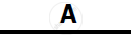conc. H2SO4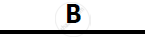2 NH3 gas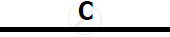Mg(OH)2 NH3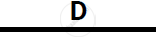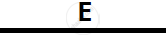Concept: Hydrogen Chloride - Preparation of Hydrogen Chloride from Sodium Chloride
Chapter: [0.083] Hydrogen Chloride
4.2

Write balanced chemical equations to show : The oxidizing action of conc. Sulphuric acid on carbon

Concept: Sulphuric Acid - Oxidizing Agent When Concentrated
Chapter: [0.084] Sulphuric Acid

Write balanced chemical equations to show The behavior of H2SO4 as an acid when it reacts with Magnesium

Concept: Hydrogen Chloride - Acidic Properties of Its Solution
Chapter: [0.083] Hydrogen Chloride

Write balanced chemical equations to show The dehydrating property of conc. Sulphuric acid with sugar.

Concept: Sulphuric Acid - Dehydrating Agent
Chapter: [0.084] Sulphuric Acid
4.3

Write balanced chemical equations to show how SO3 is converted to Sulphuric acid in the contact process.

Concept: Non-volatile Nature of Sulphuric Acid
Chapter: [0.084] Sulphuric Acid
Advertisement Remove all ads
5
5.1

Propane burns in air according to the following equation:

C3H8 + 5O2 → 3CO2 + 4H2O

What volume of propane is consumed on using 1000 cm3 of air, considering only 20% of air contains oxygen?

Concept: Gay Lussac’s Law of Combining Volumes
Chapter: [0.05] Mole Concept and Stoichiometry

The mass of 11.2 litres of a certain gas at s.t.p. is 24 g. Find the gram molecular mass of the gas

Concept: Molar Volume of a Gas at S.T.P
Chapter: [0.05] Mole Concept and Stoichiometry
5.2

A gas cylinder can hold 1 kg of hydrogen at room temperature and pressure

1) Find the number of moles of hydrogen present.

2) What weight of CO2 can the cylinder hold under similar conditions of temperature and pressure? (H = 1, C = 12, O = 16)

3) If the number of molecules of hydrogen in the cylinder is X, calculate the number of CO2 molecules in the cylinder under the same conditions of temperature and pressure.

4) State the law that helped you to arrive at the above result.

Concept: Mole Concept
Chapter: [0.05] Mole Concept and Stoichiometry
5.3

Write a balanced chemical equation for the preparation of the following salts

Copper carbonate

Concept: Preparation - Laboratory Preparation of Salts (Normal and Acid Salts) – Relevant Laboratory
Chapter: [0.03] Study of Acids, Bases and Salts

Write a balanced chemical equation for the preparation of the following salts

Ammonium sulphate crystals

Concept: Ammonia - Laboratory Preparation
Chapter: [0.081] Ammonia
6
6.1

Give a balanced chemical equation for Action of conc. Nitric acid on Sulphur

Concept: Nitric Acid as an Oxidizing Agent.
Chapter: [0.08199999999999999] Nitric Acid

Give a balanced chemical equation for Catalytic oxidation of Ammonia

Concept: Catalytic Oxidation of Ammonia as the Source of Nitric Acid
Chapter: [0.081] Ammonia

Give a balanced chemical equation for Laboratory preparation of Nitric acid.

Concept: Nitric Acid - Laboratory Method of Preparation of Nitric Acid from Potassium Nitrate Or Sodium Nitrate
Chapter: [0.08199999999999999] Nitric Acid

Give a balanced chemical equation for Reaction of Ammonia with Nitric acid

Concept: Catalytic Oxidation of Ammonia as the Source of Nitric Acid
Chapter: [0.081] Ammonia
6.2

Identify the term or substance based on the descriptions given below

Ice like crystals formed on cooling an organic acid sufficiently.

Concept: Carboxylic Acids - Concept of Acetic Acid
Chapter: [0.09] Organic Chemistry

Identify the term or substance based on the descriptions given below:

Hydrocarbon containing a triple bond used for welding purposes.

Concept: Structure of Compounds with Single, Double and Triple Bonds
Chapter: [0.09] Organic Chemistry

Identify the term or substance based on the descriptions given below:

The property by virtue of which the compound has the same molecular formula but different structural formulae.

Concept: Deduction of Simple (Empirical) and Molecular Formula from the Percentage Composition of a Compound
Chapter: [0.05] Mole Concept and Stoichiometry

The compound formed where two alkyl groups are linked by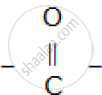group.

Concept: Organic Chemistry - Hydrocarbons - Alkynes
Chapter: [0.09] Organic Chemistry
6.3

Give a balanced chemical equation for Preparation of ethane from Sodium propionate

Concept: Alcohols - Concept of Ethanol
Chapter: [0.09] Organic Chemistry

Give a balanced chemical equation for Action of alcoholic KOH on bromethane

Concept: Alcohols - Concept of Ethanol
Chapter: [0.09] Organic Chemistry
7
7.1

Name the following :

The process of coating of iron with zinc

Concept: Electroplating
Chapter: [0.06] Electrolysis

Name the following:

An alloy of lead and tin that is used in electrical circuits.

Concept: Electrolysis - Electrolytes and Non-Electrolytes
Chapter: [0.06] Electrolysis

Name the following:

An ore of zinc containing its sulphide.

Concept: Periodic Properties - Common Ores of Iron, Aluminium and Zinc
Chapter: [0.07] Metallurgy

Name the following:

A metal oxide that can be reduced by hydrogen.

Concept: Reduction of Metallic Oxides
Chapter: [0.07] Metallurgy
7.2

Answer the following questions with respect to the electrolytic process in the extraction of aluminum:

Identify the components of the electrolyte other than pure alumina and the role played by each

Concept: Extraction of Aluminium
Chapter: [0.07] Metallurgy

Answer the following questions with respect to the electrolytic process in the extraction of aluminum:

Explain why powdered coke is sprinkled over the electrolytic mixture.

Concept: Extraction of Aluminium
Chapter: [0.07] Metallurgy
Advertisement Remove all ads
7.3

Complete the following by selecting the correct option from the choices given:

The metal which does not react with water or dilute H2SO4 but reacts with concentrated H2SO4 is _________. (Al/Cu/Zn/Fe)

Concept: Hydrogen Chloride - Acidic Properties of Its Solution
Chapter: [0.083] Hydrogen Chloride

Complete the following by selecting the correct option from the choices given:

The metal whose oxide, which is amphoteric, is reduced to metal by carbon reduction ____________. (Fe/Mg/Pb/Al)

Concept: Reduction of Metallic Oxides
Chapter: [0.07] Metallurgy

Complete the following by selecting the correct option from the choices given:

The divalent metal whose oxide is reduced to metal by electrolysis of its fused salt is __________. (Al/Na/Mg/K)

Concept: Acids, Bases and Salts as Electrolytes
Chapter: [0.06] Electrolysis
Advertisement Remove all ads

#### Request Question Paper

If you dont find a question paper, kindly write to us

View All Requests

#### Submit Question Paper

Help us maintain new question papers on Shaalaa.com, so we can continue to help students

only jpg, png and pdf files

## CISCE previous year question papers Class 10 Chemistry with solutions 2016 - 2017

CISCE Class 10 Chemistry question paper solution is key to score more marks in final exams. Students who have used our past year paper solution have significantly improved in speed and boosted their confidence to solve any question in the examination. Our CISCE Class 10 Chemistry question paper 2017 serve as a catalyst to prepare for your Chemistry board examination.
Previous year Question paper for CISCE Class 10 Chemistry-2017 is solved by experts. Solved question papers gives you the chance to check yourself after your mock test.
By referring the question paper Solutions for Chemistry, you can scale your preparation level and work on your weak areas. It will also help the candidates in developing the time-management skills. Practice makes perfect, and there is no better way to practice than to attempt previous year question paper solutions of CISCE Class 10.

How CISCE Class 10 Question Paper solutions Help Students ?
• Question paper solutions for Chemistry will helps students to prepare for exam.
• Question paper with answer will boost students confidence in exam time and also give you an idea About the important questions and topics to be prepared for the board exam.
• For finding solution of question papers no need to refer so multiple sources like textbook or guides.
Advertisement Remove all ads
Share
Notifications

View all notifications

Forgot password?
Course